Math Concepts
What is symmetry in math? Types of Symmetry and Examples
13k views

 1 Introduction 2 Types of Symmetry 3 Line of Symmetry 4 Example Problems 5 Learning Outcomes 6 About Cuemath 7 Frequently Asked Questions (FAQs) 8 External References

28 January 2021

## Introduction

Symmetry implies that one shape becomes exactly just like the other after we move it in any way i.e., turn, flip or slide. The word “Symmetry” comes from the Greek word which implies “to measure together”.

The two objects are claimed to be symmetrical, if they have the identical size and shape with one object having a different orientation from the first.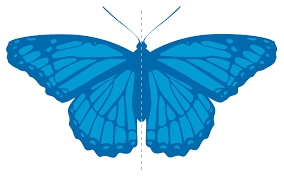The arrow of the time says that everything is moving from the highest possible symmetry to the most likely outcome. Here is a video below that explains about Symmetry, please do watch it.

### What is symmetry in math? Types of Symmetry and Examples-PDF

Symmetry implies that one shape becomes exactly just like the other after we move it in any way. Symmetry is an interesting and important topic in mathematics. Here is a downloadable PDF to explore more.

 📥 What is symmetry in math? Types of Symmetry and Examples-PDF Download

## Types of Symmetry

There are four types of symmetry. They are:

### 1. Translational Symmetry

Translational Symmetry is a type of symmetry that a figure or an image that matches exactly onto the original when it is translated at a given distance at a given direction.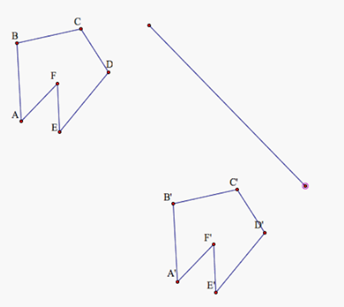### 2. Rotational Symmetry

Rotational Symmetry is a type of symmetry in which the shape or an image looks exactly similar to the original shape or image after some rotation. Rotational Symmetry is also called Radial Symmetry.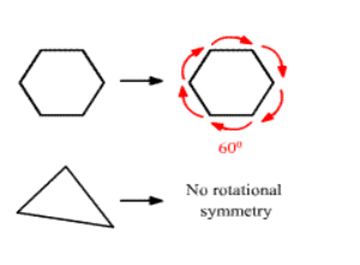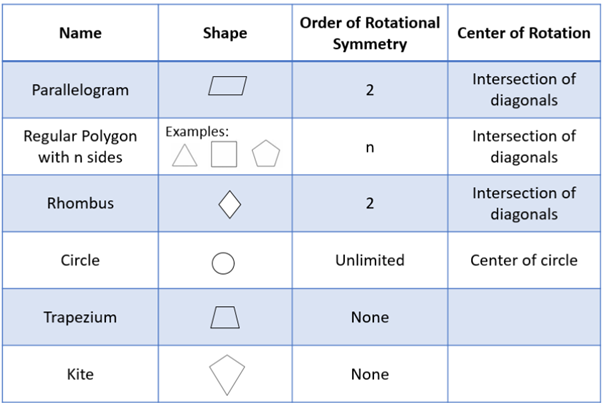### 3. Reflectional Symmetry

Reflectional Symmetry is a type of symmetry where one half of the image or picture is the reflection of the other half. Reflectional Symmetry can also be called Line Symmetry or Mirror Symmetry.A bird is reflected around a central horizontal axis.

### 4. Glide Reflection Symmetry

Glide Reflection Symmetry is a type of symmetry where the figure or image looks exactly the original when it is reflected over a line and then translated at a given distance at a given direction.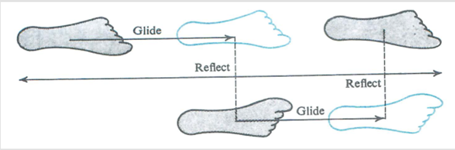The footprints trail is one of the best examples for Glide Reflection Symmetry.

## Line of Symmetry

The line of symmetry is an imaginary line or axis that passes through the centre of any picture, shape or object and it is divided into two identical halves.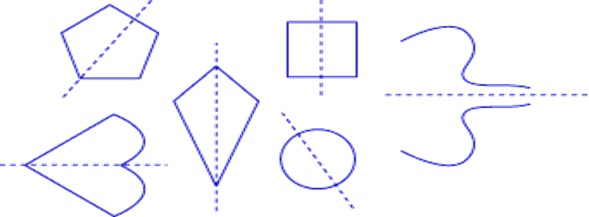Line of Symmetry of the English Alphabets

English Alphabets with vertical line of symmetry: A, H, I, M, O, T, U, V, W, X, Y.
English Alphabets with horizontal line of symmetry: B, C, D, E, H, I, K, O, X
English Alphabets with no line of symmetry: F, G, J, L, N, P, Q, R, S, Z.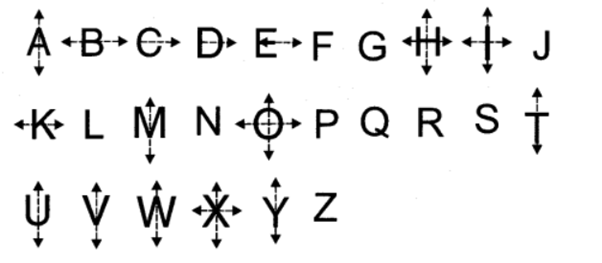## Example Problems

1. Find the order of rotation of given alphabets.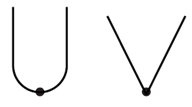(a) 1, 1
(b) 2,1
(c) 3,2
(d) 4,2

Answer: The order of rotation is 1 for both alphabets as it is reflection symmetry about a vertical line at the centre. The answer is Option (a).

 (a) 1, 1

2. What is the least number of squares that must be added so that the line AB becomes the line of symmetry?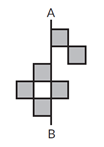(a) 4
(b) 5
(c) 6
(d) 7

 (c) 6

3. Which of the following letters of English Alphabets have more than 2 lines of Symmetry?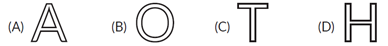Answer:  The answer is option (B) as Alphabet ‘A’ and ‘T’ has one line of symmetry and Alphabet ‘H’ has 2 lines of symmetry. Only Alphabet ‘O’ has an infinite number of lines of Symmetry.

 (B) O

4. After rotating the figure by 15° about its centre, it looks the same as its original position. At which another angle will this happen again?
(a) 40°
(b) 60°
(c) 20°
(d) 80°

Answer: The answer is option (b). The figure is rotated 15°. It looks absolutely the same during the multiples of 15. Therefore, 15*4=60.

 (b) 60°

5. A parallelogram has ------------------
(a) No line of Symmetry
(b) One line of Symmetry
(c) Two line of Symmetry
(d) Four line of SymmetryThe diagonals of the parallelogram are the line of Symmetry. Therefore, the parallelogram has no line of Symmetry.

 (a) No line of Symmetry

6. The number of English Alphabets (Capital) that do not have a line of symmetry is ---------------
(a) 9
(b) 10
(c) 11
(d)12

Answer: The answer is option (c). The English Alphabet which has zero lines of symmetry are F,G,J,K,L, N,P,Q,R,S,Z.

 (c) 11

7. Which of the following statements are false?
(a) A figure can have angle of rotational symmetry is 100°
(b) I and H are the only letters that have two lines of symmetry.
(c) An equilateral triangle has only 3 lines of symmetry.
(d) None of these

Answer: The answer is option (b). There are 4 English Alphabet which have two lines of symmetry.

 (b) I and H are the only letters that have two lines of symmetry

8. How many lines of symmetry does an isosceles triangle have?
(a) 0
(b) 1
(c) 2
(d) 3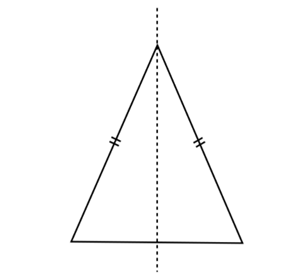(b) 1

9. Which of the following letters does not have a line of symmetry but has a rotational symmetry?
(a) H
(b) I
(c) Z
(d) X

Answer:  The answer is option (c). Z does not have a line of symmetry. But it has only rotational symmetry.

 (c) Z

10. The order of rotational symmetry of a circle is ------------------
(a) 0
(b) 1
(c) 2
(d) Infinite

 (d) Infinite

## Learning Outcomes

• We learnt how to draw lines of symmetry for various figures.
• We learnt how to analyze the figures of rotational symmetry by rotating them.
• After going through this blog, practice more problems.

Cuemath, a student-friendly mathematics platform, conducts regular Online Classes for academics and skill-development, and their Mental Math App, on both iOS and Android, is a one-stop solution for kids to develop multiple skills. Understand the Cuemath Fee structure and sign up for a free trial.

## What is the use of Symmetry?

Symmetry is used in Physics to understand the properties of the molecules.  It is used in understanding the mirror image of the molecules by any combinations of translations and rotations.

## Why is Symmetry important?

Symmetry helps us to organize things in a better manner. According to Physics, Translation Symmetry helps to conserve the energy and momentum.

## What is Bilateral Symmetry and Point Symmetry?

Bilateral Symmetry is a symmetry where the figure is divided into two equivalent right and left halves by only one plane. We can observe bilateral Symmetry in plants and Animals.

Point Symmetry is when each and every part of a figure or image has an exact match but in the opposite direction.

-Written by Anusha Makam D, Cuemath Teacher

Related Articles